* how to use the cards * print the x ÷ cards * x ÷ games and activities
* How to use the fact family cards
Each card has three numbers on it. The number at the bottom is the product of the two numbers above it. These three numbers are a fact family. This card shows four related facts: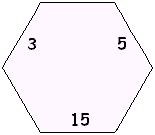3 x 5 = 15
5 x 3 = 15
15 ÷ 3 = 5
15 ÷ 5 = 3
Cut and glue a "fact family house" to hide one number at a time.
Place a stack of fact family cards in the house, say the missing number on the card (see the examples below), then pull the card off the stack and go on to the next card. At any time, you can move the position of the house so that it is hiding a different side of the cards.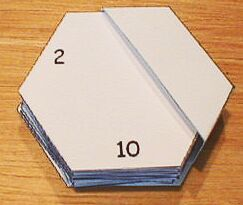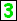x 5 = 15What times 5 is 15?15 ÷ 5 =What is 15 ÷ 5?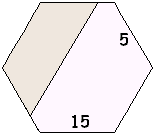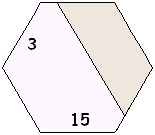3 x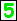= 153 times what is 15?15 ÷ 3 =What is 15 ÷ 3?3 x 5 =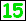3 times 5 is what?÷ 3 = 5 What ÷ 3 = 5?÷ 5 = 3 What ÷ 5 = 3?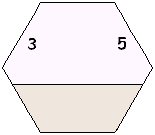You can even place the house so that its roof hides the top two numbers, then factor the number you can see: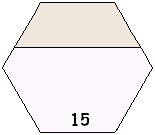x= 15x= 15 15 ÷=15 ÷=Sometimes the bottom number can be factored in different ways. For instance, 12 could be 3 x 4, 4 x 3, 2 x 6, or 6 x 2. You can turn this situation into a guessing game. See the activities below.